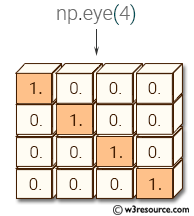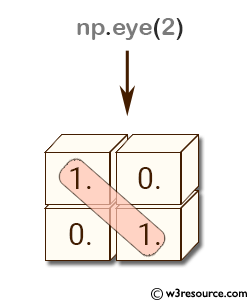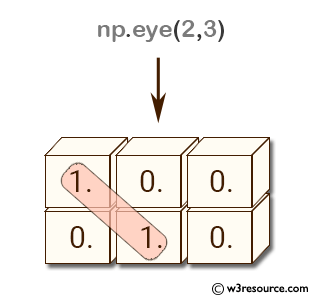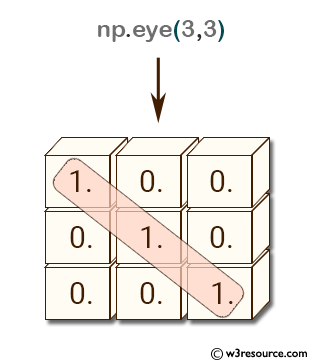# NumPy: numpy.eye() function

## numpy.eye() function

The eye() function is used to create a 2-D array with ones on the diagonal and zeros elsewhere.
The eye() function is commonly used in linear algebra and matrix operations. It is useful for generating matrices to transform, rotate, or scale vectors. It can also be used in scientific computing for solving differential equations, optimization, and signal processing.

Syntax:

`numpy.eye(N, M=None, k=0, dtype=<class 'float'>, order='C')`Parameters:

Name Description Required /
Optional
N Number of rows in the output. Required
M Number of columns in the output. If None, defaults to N. optional
k Index of the diagonal: 0 (the default) refers to the main diagonal, a positive value refers to an upper diagonal, and a negative value to a lower diagonal. optional
dtype Data-type of the returned array. optional
order Whether the output should be stored in row-major (C-style) or column-major (Fortran-style) order in memory optional

Return value:

[ndarray of shape (N,M)] An array where all elements are equal to zero, except for the k-th diagonal, whose values are equal to one.

Example-1: Identity Matrix using NumPy eye function

``````>>> import numpy as np
>>> np.eye(2)
array([[ 1.,  0.],
[ 0.,  1.]])
>>> np.eye(2,3)
array([[ 1.,  0.,  0.],
[ 0.,  1.,  0.]])
>>> np.eye(3, 3)
array([[ 1.,  0.,  0.],
[ 0.,  1.,  0.],
[ 0.,  0.,  1.]])
>>> ``````

In linear algebra, an identity matrix is a square matrix with ones on the main diagonal and zeros elsewhere.

In the first example, np.eye(2) creates a 2x2 identity matrix where both the rows and columns are equal to 2.

In the second example, np.eye(2,3) creates a 2x3 identity matrix where the first argument specifies the number of rows and the second argument specifies the number of columns.

In the third example, np.eye(3,3) creates a 3x3 identity matrix where both the rows and columns are equal to 3.

Pictorial Presentation:Example-2: Identity Matrix using NumPy eye function

``````>>> import numpy as np
>>> np.eye(2, dtype=int)
array([[1, 0],
[0, 1]])
>>> np.eye(2,2,  dtype=int)
array([[1, 0],
[0, 1]])
>>> np.eye(2,2,  dtype=float)
array([[ 1.,  0.],
[ 0.,  1.]])
>>> ``````

In the first example, np.eye(2, dtype=int) creates a 2x2 identity matrix with integer data type. The resulting array is array([[1, 0], [0, 1]]).

In the second example, np.eye(2,2, dtype=int) creates the same 2x2 identity matrix with integer data type. The 2,2 parameter specifies the size of the matrix, and since the dtype parameter is set to int, the resulting array is the same as the first example.

In the third example, np.eye(2,2, dtype=float) creates a 2x2 identity matrix with float data type. The resulting array is array([[1., 0.], [0., 1.]])

Example: Create a sparse identity matrix with a non-zero diagonal offset:

Code:

``````import numpy as np

# Create a sparse identity matrix with diagonal offset of 1
nums = np.eye(5, k=1, dtype=int)
print(nums)

``````

Output:

```[[0 1 0 0 0]
[0 0 1 0 0]
[0 0 0 1 0]
[0 0 0 0 1]
[0 0 0 0 0]]
```

In the above example, we create a sparse identity matrix with dimensions (5, 5) and a diagonal offset of 1. This means that the diagonal elements are shifted one position to the right, resulting in a matrix with 1's on the first upper diagonal and 0's elsewhere.

Example: Create a complex-valued identity matrix with non-unit diagonal elements

Code:

``````import numpy as np

# Create a complex-valued identity matrix with diagonal elements of 1+1j
nums = np.eye(3, dtype=complex) * (1 + 1j)
print(nums)

``````

Output:

```[[1.+1.j 0.+0.j 0.+0.j]
[0.+0.j 1.+1.j 0.+0.j]
[0.+0.j 0.+0.j 1.+1.j]]
```

In the above example, we create a complex-valued identity matrix with dimensions (3, 3) and diagonal elements of 1+1j (i.e., the real part is 1 and the imaginary part is 1). In order to achieve this, we multiply the output of np.eye() function by the complex scalar (1+1j). The resulting matrix has diagonal elements of 1+1j and 0's elsewhere.

Python - NumPy Code Editor:

Previous: empty_like()
Next: identity()

﻿